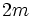# Linear representation theory of dicyclic groups

This article gives specific information, namely, linear representation theory, about a family of groups, namely: dicyclic group.
View linear representation theory of group families | View other specific information about dicyclic group

This article discusses the linear representation theory of dicyclic groups. The dicyclic group of order$4n$, and degree$n$, is given by the following presentation:$\langle a,x \mid a^{2n} = e, x^2 = a^n, x^{-1}ax = a^{-1} \rangle$.

For$n \ge 2$, the center of the dicyclic group is the two-element subgroup generated by$a^n$, and the quotient by that is the dihedral group of degree$n$ and order$2n$.

It turns out that the character tables of dicyclic group of degree$2m$ (and hence, order$8m$) and the dihedral group of degree$4m$ (and hence, order$8m$) are equivalent. Further information: Dicyclic group of even degree has the same character table as dihedral group of same order

## The linear representation theory of dicyclic groups of even degree

### Representations arising from the dihedral group

Since there are$n + 3$ conjugacy classes of elements, there are$n+3$ irreducible representations over the complex numbers. Of these,$(n+6)/2$ irreducible representations arise from irreducible representations of the quotient by the center, which is the dihedral group of degree$n$. Specifically, for any irreducible representation of the dihedral group of degree$n$, we compose with the quotient map to obtain an irreducible representation of the dicyclic group of degree$n$.

This includes four one-dimensional representations and$(n-2)/2$ two-dimensional representations.

For a complete description of these$(n + 6)/2$ irreducible representations, refer linear representation theory of dihedral groups#the linear representation theory of dihedral groups of even degree.

### Other representations

There are$n/2$ other irreducible representations of degree two. These are parametrized by the odd integers from$1$ to$n$. Specifically, for$k = 1,2, \dots, n - 1$, define the representation:$a \mapsto \begin{pmatrix} e^{\pi ik/n} & 0 \\ 0 & e^{-i\pi k/n} \\\end{pmatrix}, \qquad x \mapsto \begin{pmatrix} 0 & -1 \\ 1 & 0 \\\end{pmatrix}$.

Note that in all these representations, the non-identity central element$a^n$ is mapped to the negative of the identity matrix. All these representations are quaternionic representations. Specifically, they arise from homomorphisms from the dicyclic group to the multiplicative group of Hamiltonian quaternions.

Note that we also get representations for$k = n+1, n +3, \dots, 2n - 1$, but the representation for$k$ is equivalent to the representation for$2n- k$.

## The linear representation theory of dicyclic groups of odd degree

### Representations arising from the dihedral groups

Since there are$n + 3$ conjugacy classes, there are$n + 3$ irreducible representations over the complex numbers. Of these,$(n+3)/2$ irreducible representations arise from irreducible representations of the quotient by the center, which is the dihedral group of degree$n$. Specifically, for any irreducible representation of the dihedral group of degree$n$, we compose with the quotient map to obtain an irreducible representation of the dicyclic group of degree$n$.

This includes two one-dimensional representations and$(n-1)/2$ irreducible two-dimensional representations.

For a complete description of these$(n + 6)/2$ irreducible representations, refer linear representation theory of dihedral groups#the linear representation theory of dihedral groups of odd degree.

### Other one-dimensional representations

There are two more one-dimensional representations that do not have the center in their kernel. TO obtain these representations, note that the commutator subgroup is$\langle a^2 \rangle$, and the abelianization is a cyclic group of order four generated by the image of$x$. Since$n$ is odd,$a^n$ lies outside the commutator subgroup. There are two one-dimensional representations with kernel$\langle a^2 \rangle$:

• The representation sending the coset of$x$ to$(i)$, the coset of$a^n$ to$(-1)$, and the coset of$ax$ to$(-i)$.
• The representation sending the coset of$x$ to$(-i)$, the coset of$a^n$ to$(-1)$, and the coset of$ax$ to$(i)$.

### Other two-dimensional representations

There are$(n-1)/2$ other irreducible representations of degree two. These are parametrized by the odd integers from$1$ to$n - 2$. Specifically, for$k = 1,2, \dots, n - 2$, define the representation:$a \mapsto \begin{pmatrix} e^{\pi ik/n} & 0 \\ 0 & e^{-i\pi k/n} \\\end{pmatrix}, \qquad x \mapsto \begin{pmatrix} 0 & -1 \\ 1 & 0 \\\end{pmatrix}$.

Note that in all these representations, the non-identity central element$a^n$ is mapped to the negative of the identity matrix. All these representations are quaternionic representations. Specifically, they arise from homomorphisms from the dicyclic group to the multiplicative group of Hamiltonian quaternions.

Note that we also get representations for$k = n+2, n + 4, \dots, 2n - 1$, but the representation for$k$ is equivalent to the representation for$2n- k$.

## Orthogonality relations and numerical checks

• In both the odd and even cases, there are$4$ one-dimensional representations and$n - 1$ irreducible two-dimensional representations. Thus, the number of irreducible representations equals the number of conjugacy classes:$n + 3$.
• In both cases, the sum of squares of degrees of irreducible representation is$4(1)^2 + (n-1)(2)^2 = 4n$, which is the order of the group. This confirms the fact that sum of squares of degrees of irreducible representations equals order of group.
• In both cases, all representations have degree$1$ or$2$. This is consistent with the fact that there is an abelian normal subgroup$\langle a \rangle$ of index two, and degree of irreducible representation divides index of abelian normal subgroup.
• In the case of even degree, all the representations have real-valued characters. this is consistent with the fact that the dicyclic groups are ambivalent groups: every element is conjugate to its inverse.